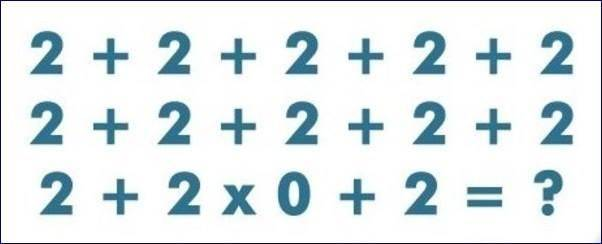### How Quick Can You Solve This Maths Puzzle?

Answer this maths puzzle as quickly as possible and tell us the answer you got in your first attempt. DO Not Cheat!#### Related Posts

###### NEKEDEPOLY Notice To Students On Payment of School Fees, 2019/2020

24
Follow
•      1 year ago
•      1 year ago
• Omila2016: I learnt this by reading a blog post on how to created love for maths...
Just check it out wen u are free, its actually helpful..
https://enterschools.com/how-to-create-love-for-maths-2/
1 year ago
• Iheanyi: 24
1 month ago
•      1 month ago
• Solomon: 2
1 month ago
• sir nature: The answer is = 2
1 month ago
•      1 month ago
• Leah: 24
1 month ago
• Philip: 24
1 month ago
• Rachael: The answer is 2
1 month ago
• Adeyemo: the answe is 2
1 month ago
• Ify: The answer is 2
1 month ago
•      1 month ago
• Muizdino: 24
1 month ago
•      1 month ago
1 month ago
•      1 month ago
•      1 month ago
• olajuwon: 2 is the answer
1 month ago
•      1 month ago
•      1 month ago
•      1 month ago
• Genksoul: Solution:
Applying BODMAS
2 in 12places=2*12 which 24
Also any value multiply by zero is nothing
Therefore, 24*0=0
Then 0+2= 2
1 month ago
• Feranmi: 2
1 month ago
• Hannah: 24
1 month ago
• OjieOJ: The answer is 24
Multiply 2 by the zero beside it and then sum the remain 22
1 month ago
• shotanny: The answer is 4
1 month ago
• Ifeanyi: The answer is 2
1 month ago
• IhiMa: 2
1 month ago
• Nkeoma: 1½
1 month ago
• Nkeoma: 24
1 month ago
• Chioma Orji: 24 is the answer
1 month ago
• Calista: 2*12 =24
24*0+2 = 2
Ans: 2
1 month ago
•      1 month ago
•      1 month ago
• Oliver: 2
1 month ago
• Damirichie: The answer is 2
1 month ago
•      1 month ago
•      1 month ago
• Gift Ese: Multiplication comes before addition hence 24 is most Correct.
3 weeks ago
• Egbon Vanessa: the answer is 2
1 week ago
•      1 week ago
•      1 week ago
• Emmanuel moses: 24*0=0 so the ans is 2
1 week ago
24
Follow
• Mujeeb: 24
1 month ago
• Abdul: 2
1 month ago
• Calista: 2
1 month ago
• Melody: 2
2 weeks ago
•      2 weeks ago
• princess: the answer is 2
24*0=0
0+2=21 week ago
• princess: the answer is 2
24*0=0
0+2=2
1 week ago
• David123: 24
1 week ago
• Ella: 2
1 week ago
24
Follow
Follow
Follow
• PETER: 24
1 month ago
Follow
2
Follow
24
Follow
Therefore 2+2+2+2+2
2+2+2+2+2
2+2×0+2=?
Follow
• Ukah P: 2+(2*0)+2
=2+0+2=4
1 month ago
26
Follow
Follow
24
Follow
2+2+2+2+2+......multiply by 0=0 and 0 multiply by 2=2 so the answer is 2
Follow
Follow
24
Follow

#### Quick Questions

• 0 Answers   ·   44 minutes ago
• 0 Answers   ·   1 hour ago
• 1 Answers   ·   2 hours ago

#### Post Comment

Please don't post or ask to join a "Group" or "Whatsapp Group" as a comment. It will be deleted. To join or start a group, please click here

##### Marketplace
• Here are the things students around you have to sell;
• ###### Tecno t528
₦1,500   FCAA   Used